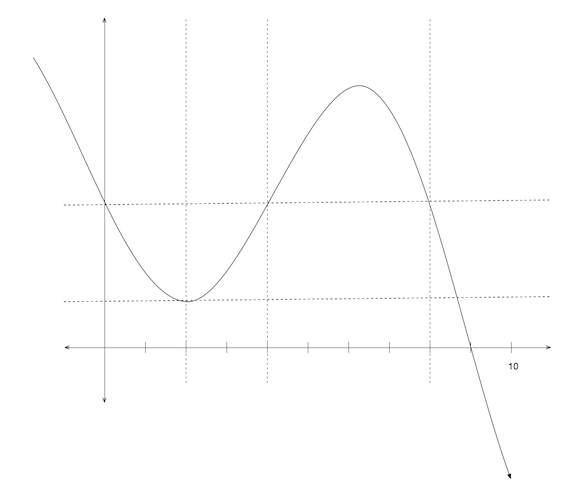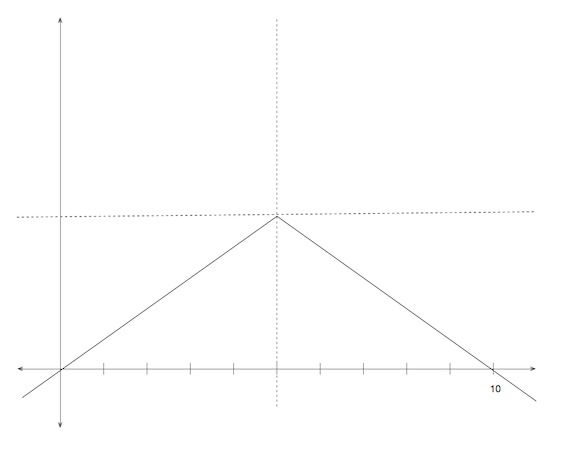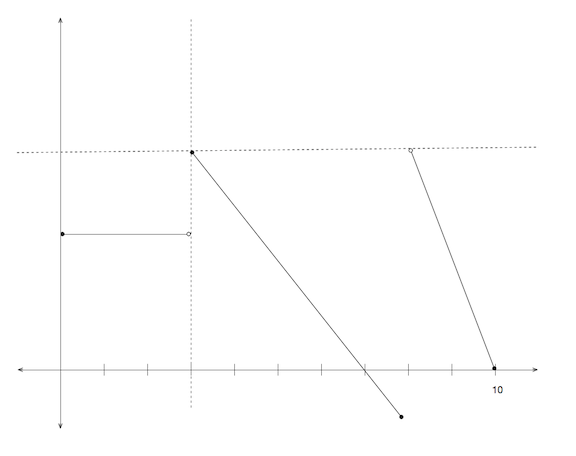Algebra

Interpreting Graphs of FunctionsIn the above graph, if $f(0) = 7$, what is the value of $f( 4)$?The above graph consists of 2 straight lines. If $f( 5) = 20$, what is the value of $f( 6 )$?The above graph consists of 2 straight lines. If $f(1 ) = 5$, what is the value of $f( 9 )$?The above graph represents an algebraic function that is defined on the domain $[0, 10]$. If $f( 2) = 3$ and $f(3) = 5$, how many solutions are there to $f(x) = 1$?In the above graph, if $f(0) = 3$, what is the value of $f(9)$?

×